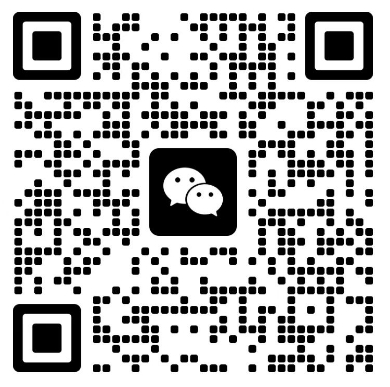# 全国2007年4月自学考试计算机基础与程序设计试题

1．I/O设备指的是计算机的（　　　）
A．存储设备 B．输入和输出设备 C．控制器 D．运算器
2．具有只读功能的内存储器是指（　　　）
A．ROM B．RAM C．硬盘 D．CD-ROM
3．十进制数－5在八位的微机内的存储形式是（　　　）
A．00000101 B．10000101 C．11111010 D．11111011
4．C语言源程序的基本单位是（　　　）
A．过程  B．函数 C．子程序 D．语句
5．设有int p，q；以下不正确的语句是（　　　）
A．p *=3； B．p/=q； C．p+=3； D．p&&=q；
6．若有下列程序段，欲使a=2，b=3，对应正确的数据输入方法是（其中<CR>表示换行）
（　　　）
int a，b
scanf(＂%d%d＂，&a，&b)；
A．2<CR> B．a=2  b=3<CR>
3<CR>
C．a=2，b=3<CR> D．2，3<CR>
7．设有说明 int x=0,y=2;

else y=x-1;

A．-1 B．0 C．1 D．2
8．下面程序的输出结果是（　　　）
main(  )
{
int x=5,y;
if(x>0)
y=1;
y=0;
if(x<0)
y=-1;
printf(＂%d＂,y);
}
A．-1 B．0 C．1 D．5
9．执行下面程序段后，输出字符B的个数是（　　　）
x=0;
while(x<=5){
printf(＂B＂);
x+=2;
}
A．0个 B．3个 C．5个 D．6个
10．在下述程序中，for循环执行的次数是（　　　）
main(  )
{
int i =0,j=10,k=2,s=0;
for(; ;)
{
i+=k;
if(i>j)
{
printf(＂%d\n＂,s);
break;
}
s+=i;
}
}
A．4 B．5 C．6 D．7
11．若有定义：int a；则数组元素的下标的下限和上限分别是（　　　）
A．0和1 B．1和9 C．0和9 D．1和10
12．下列说法不正确的是（　　　）
A．一个源程序文件由一个或多个函数组成
B．一个C程序由一个或多个源程序文件组成
C．一个C程序以函数为独立的编译单位
D．一个C程序必须有一个main( )函数
13．在C语言中，确定函数返回值的类型由（　　　）
A．return语句中的表达式类型决定 B．调用该函数的主函数类型决定
C．调用函数时临时决定 D．定义函数时所指定的函数类型决定
14．若有以下定义和语句，且0≤i＜10，则对数组元素地址的正确表示是（　　　）
int a [ ]={0,1,2,3,4,5,6,7,8,9},*p;
p=a;
A．&(a+1) B．a++ C．&p D．&p[i]
15．若有以下说明：
char s=“ABCDEFGH”,*p=s;
不能表示字符C的是（　　　）
A．s B．s C．s[＇c＇－＇a＇] D．*(p+2)
16．有下列程序：
func(int x, int y, int*jia, int*jian)
{*jia=x+y;
*jian=x-y;}
main( )
{int i =10,j=3,x1,x2;
func( i ,j,&x1,&x2 );
printf(＂%d,&d\n＂,x1,x2);}

A．0，0 B．10，3 C．13，7 D．7，13
17．设有结构体的定义如下：
struct data{
int n;
char c;
float f;
};

A．1 B．3 C．7 D．11
18．如果在程序中要使用系统提供的函数sqrt，则需要书写的编译预处理命令是（　　　）
A．#include<stdio. h>  B．#include<math. h>
C．#include<string.h>  D．#include<malloc.h>
19．设有说明：int x=12,y=3;则x￤y的值是（　　　）
A．0 B．3 C．12 D．15
20．测试文件是否结束函数的函数名是（　　　）
A．feof B．EOF C．eof D．FEOF

21．下列设备中属于外存储设备的有（　　　　　）
A．RAM B．光盘 C．硬盘
D．软盘 E．磁带
22．设a=1,b=3,c=0；下面表达式的值为1的有（　　　　　）
A．!a&&b| |a&&(c+1) B．a－b+3 C．(a>b)==c
D．(a=0)&&(b=10) E．b>a&&a>c
23．设有说明：char s；（　　　　　）
下面各项中能够正确的将一个字符串＂abcd＂赋给s数组的有
A．s=＂abcd＂; B．gets(s); C．scanf(＂%s＂,s);
D．strcpy(s,＂abcd＂); E．strcpy(＂abcd＂,s);
24．关于结构体，下面各项中不正确的有（　　　　　）
A．struct person{long bh; char *name; int age;}
psl={9100,＂wang＂,20},* pst;
pst=psl; printf(＂%d＂,*pst→age);
B．struct my_data
{int num; struct my_data *next;};
C．struct test
{char *course; char *class}
D．struct worker
{char name; float gz;}Li={＂Li Ping＂,532.67};
E．struct int_num
{int i _num;struct int_num *next; }ione, *pi;
pi=&ione;
25．设有说明：int x=1,y=2,z=3；则下面表达式的值为0的有（　　　　　）
A．x&y&z B．x￤y￤z C．x^x+y^y
D．z>>y E．x&0xff00

26．C语言中的运算符“=”与运算符“= =”的意义相同吗？为什么？
27．变量的存储类别分为哪几种？它们分别存储在什么区域？
28．试定义一个三行的二维字符数组s，并用＂I am a boy＂, ＂you are a girl＂, ＂he is a teacher＂对其进行初始化。
29．设有说明：int a；
请用四种方式来表示数组元素a。

30．从键盘输入字符A时，写出下面程序的运行结果。（注：字符A的ASCII码是65）
#include＂stdio.h＂
main( )
{
char ch;
ch=putchar(getchar( ) );
printf(＂,%d,%o,%c＂,ch+1,ch+1,ch+32);
}
31．阅读下面程序：
void main(void)
{
int s=0,n=0,x;
label: scanf(＂%d＂,&x);
if(x>0){
s+=x;
n++;
printf(＂s=%d,n=%d,even=%f\n＂,s,n,1.0,*s/n);
}
if(x!=0)
goto label;
else
printf(＂good bye!＂);
}

32．阅读下面程序，写出程序的运行结果。
char a [ ]={＇9＇，＇8＇，＇7＇，＇6＇，＇5＇，＇4＇，＇3＇，＇2，＇1＇，＇0＇，＇\0＇};
void main (void)
{
int i =8,j;
for(j=0;j<4;j＋＋)
printf(＂%s\n＂,&a[i －j ]);
}
33．请写出下面程序的运行结果。
long fun (int k )
{
long fac;
if(k= =0)
return 1;
else
{
fac=k* fun(k-1);
printf(＂fac=%ld\n＂,fac);
}
return fac;
}
void main(void)
{
int n=3;
printf(＂result=%ld\n＂,fun(n+1)/n);
}
34.阅读下面程序，写出运行结果。
#include<stdio.h>
#include<string.h>
void f(char *s)
{
char *t, c, *p=s;
for(t=s+strlen(s)-1;s<t;s＋＋,t――)
{
c=*s,*s=*t,*t=c;
printf(＂%s\n＂,p);
}
}
void main (void)
{
char a =＂abcdefg＂;
f(a);
}
35．阅读下面程序段，说明其功能：
int x, y=0,k; FILE* fp;
fp=fopen(＂data.txt＂,＂rb＂);
for(k=0;k<5;k++)
printf(＂%d,＂y);
fclose(fp);

36．从键盘输入10个整数并依次赋给整型数组中的元素，然后求该数组中值为正数的元素

37．在c盘根目录下有一个ASCII码文件month.dat，文件中有12个字符串分别为英文1~12

【免责声明】

1、由于各方面情况的调整与变化，本网提供的考试信息仅供参考，考试信息以省考试院及院校官方发布的信息为准。

2、本网信息来源为其他媒体的稿件转载，免费转载出于非商业性学习目的，版权归原作者所有，如有内容与版权问题等请与本站联系。联系邮箱：952056566@qq.com

### 微信公众号（请添加[客服老师微信]）